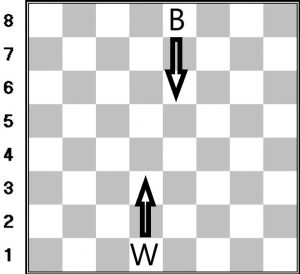# Chessboard Pawn-Pawn game

• Difficulty Level : Medium
• Last Updated : 02 Jun, 2021

There is an 8*8 chessboard and two chess players having a single pawn each. A player has to move his pawn in each turn, either one step forward or one step diagonally only when this move kills the other pawn. The player who is unable to make any move loses.
Given row and column numbers of white and black pawns. The task is to predict who would win assuming both plays optimally. Note that White plays first and a pawn cannot move outside the chessboard.Examples:

Input: rowW = 2, colW = 2, rowB = 3, colB = 3
Output: White
Input: rowW = 2, colW = 2, rowB = 3, colB = 3
Output: White

Approach:

• If its white pawn’s turn we have to check whether white pawn is on the 8th row then black wins because white pawn has no further move. If it’s black pawn’s turn then we have to check whether it is on the 1st row then white wins because black pawn has no further move.
• If its white pawn’s turn and black pawn is adjacent diagonally then white pawn will kill black pawn and white pawn wins else white pawn will move one step forward (if not already occupied by the black pawn) else white will lose.
• If it’s black pawn’s turn and white pawn is adjacent diagonally then black pawn will kill white pawn and black pawn wins else black pawn will move one step forward (if not already occupied by the white pawn) else black will lose.

Below is the implementation of the above approach:

## C++

 `// C++ implementation of the approach``#include ``using` `namespace` `std;` `// Function that returns true if white wins``bool` `whiteWins(``int` `rowW, ``int` `colW, ``int` `rowB, ``int` `colB)``{``    ``int` `white = 0, black = 0;` `    ``while` `(1) {` `        ``// If white can move``        ``if` `(rowW != 8) {` `            ``// If white pawn can kill black pawn``            ``// White wins``            ``if` `(rowB == rowW + 1``                ``&& (colB == colW - 1 || colB == colW + 1))``                ``return` `true``;` `            ``// Make the move forward``            ``else``                ``rowW++;``        ``}` `        ``// White has no moves``        ``// White loses``        ``else``            ``return` `false``;` `        ``// If black can move``        ``if` `(rowB != 1) {` `            ``// If black pawn can kill white pawn``            ``// White loses``            ``if` `(rowB == rowW + 1``                ``&& (colB == colW - 1 || colB == colW + 1))``                ``return` `false``;` `            ``// Make the move forward``            ``else``                ``rowB--;``        ``}` `        ``// Black has no moves``        ``// White wins``        ``else``            ``return` `true``;``    ``}` `    ``// If white has got more moves``    ``if` `(white > black)``        ``return` `true``;` `    ``return` `false``;``}` `// Driver code``int` `main()``{``    ``int` `rowW = 2, colW = 2, rowB = 3, colB = 3;``    ``if` `(whiteWins(rowW, colW, rowB, colB))``        ``cout << ``"White"``;``    ``else``        ``cout << ``"Black"``;``    ``return` `0;``}`

## Java

 `// Java implementation of the approach``class` `GFG``{``    ` `// Function that returns true if white wins``static` `boolean` `whiteWins(``int` `rowW, ``int` `colW,``                        ``int` `rowB, ``int` `colB)``{``    ``int` `white = ``0``, black = ``0``;``    ``boolean` `flag=``true``;` `    ``while` `(flag)``    ``{` `        ``// If white can move``        ``if` `(rowW != ``8``)``        ``{` `            ``// If white pawn can kill black pawn``            ``// White wins``            ``if` `(rowB == rowW + ``1``                ``&& (colB == colW - ``1` `|| colB == colW + ``1``))``                ``return` `true``;` `            ``// Make the move forward``            ``else``                ``rowW++;``        ``}` `        ``// White has no moves``        ``// White loses``        ``else``            ``return` `false``;` `        ``// If black can move``        ``if` `(rowB != ``1``)``        ``{` `            ``// If black pawn can kill white pawn``            ``// White loses``            ``if` `(rowB == rowW + ``1``                ``&& (colB == colW - ``1` `|| colB == colW + ``1``))``                ``return` `false``;` `            ``// Make the move forward``            ``else``                ``rowB--;``        ``}` `        ``// Black has no moves``        ``// White wins``        ``else``            ``return` `true``;``    ``}` `    ``// If white has got more moves``    ``if` `(white > black)``        ``return` `true``;` `    ``return` `false``;``}` `// Driver code``public` `static` `void` `main(String args[])``{``    ``int` `rowW = ``2``, colW = ``2``, rowB = ``3``, colB = ``3``;``    ``if` `(whiteWins(rowW, colW, rowB, colB))``        ``System.out.println(``"White"``);``    ``else``        ``System.out.println(``"Black"``);``}``}` `// This code is contributed by Arnab Kundu`

## Python3

 `# Print implementation of the approach` `# Function that returns true if white wins``def` `whiteWins(rowW, colW, rowB, colB):``    ``white ``=` `0``;``    ``black ``=` `0``;` `    ``while` `(``1``):` `        ``# If white can move``        ``if` `(rowW !``=` `8``):` `            ``# If white pawn can kill black pawn``            ``# White wins``            ``if` `(rowB ``=``=` `rowW ``+` `1` `and``               ``(colB ``=``=` `colW ``-` `1` `or``                ``colB ``=``=` `colW ``+` `1``)):``                ``return` `True``;` `            ``# Make the move forward``            ``else``:``                ``rowW ``+``=` `1``;` `        ``# White has no moves``        ``# White loses``        ``else``:``            ``return` `False``;` `        ``# If black can move``        ``if` `(rowB !``=` `1``):` `            ``# If black pawn can kill white pawn``            ``# White loses``            ``if` `(rowB ``=``=` `rowW ``+` `1` `and``               ``(colB ``=``=` `colW ``-` `1` `or``                ``colB ``=``=` `colW ``+` `1``)):``                ``return` `False``;` `            ``# Make the move forward``            ``else``:``                ``rowB ``-``=` `1``;` `        ``# Black has no moves``        ``# White wins``        ``else``:``            ``return` `Frue;` `    ``# If white has got more moves``    ``if` `(white > black):``        ``return` `True``;` `    ``return` `False``;` `# Driver code``if` `__name__ ``=``=` `'__main__'``:``    ``rowW, colW ``=` `2``, ``2``;``    ``rowB, colB ``=` `3``, ``3``;``    ``if` `(whiteWins(rowW, colW, rowB, colB)):``        ``print``(``"White"``);``    ``else``:``        ``print``(``"Black"``);` `# This code is contributed by Rajput-Ji`

## C#

 `// C# implementation of the approach``using` `System;``public` `class` `GFG``{``     ` `// Function that returns true if white wins``static` `bool` `whiteWins(``int` `rowW, ``int` `colW,``                        ``int` `rowB, ``int` `colB)``{``    ``int` `white = 0, black = 0;``    ``bool` `flag=``true``;`` ` `    ``while` `(flag)``    ``{`` ` `        ``// If white can move``        ``if` `(rowW != 8)``        ``{`` ` `            ``// If white pawn can kill black pawn``            ``// White wins``            ``if` `(rowB == rowW + 1``                ``&& (colB == colW - 1 || colB == colW + 1))``                ``return` `true``;`` ` `            ``// Make the move forward``            ``else``                ``rowW++;``        ``}`` ` `        ``// White has no moves``        ``// White loses``        ``else``            ``return` `false``;`` ` `        ``// If black can move``        ``if` `(rowB != 1)``        ``{`` ` `            ``// If black pawn can kill white pawn``            ``// White loses``            ``if` `(rowB == rowW + 1``                ``&& (colB == colW - 1 || colB == colW + 1))``                ``return` `false``;`` ` `            ``// Make the move forward``            ``else``                ``rowB--;``        ``}`` ` `        ``// Black has no moves``        ``// White wins``        ``else``            ``return` `true``;``    ``}`` ` `    ``// If white has got more moves``    ``if` `(white > black)``        ``return` `true``;`` ` `    ``return` `false``;``}`` ` `// Driver code``public` `static` `void` `Main(String []args)``{``    ``int` `rowW = 2, colW = 2, rowB = 3, colB = 3;``    ``if` `(whiteWins(rowW, colW, rowB, colB))``        ``Console.WriteLine(``"White"``);``    ``else``        ``Console.WriteLine(``"Black"``);``}``}``/* This code contributed by PrinciRaj1992 */`

## Javascript

 ``
Output:
`White`

My Personal Notes arrow_drop_up